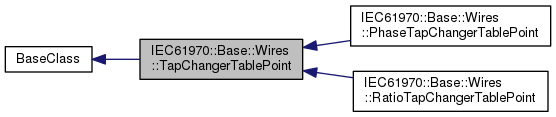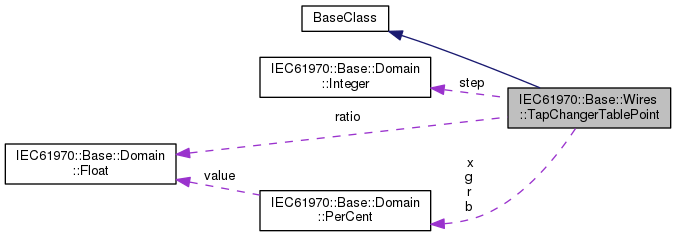IEC61970 17v07IEC61970::Base::Wires::TapChangerTablePoint Class Reference
Inheritance diagram for IEC61970::Base::Wires::TapChangerTablePoint:[legend]
Collaboration diagram for IEC61970::Base::Wires::TapChangerTablePoint:[legend]

## Public Attributes

IEC61970::Base::Domain::PerCent b

IEC61970::Base::Domain::PerCent g

IEC61970::Base::Domain::PerCent r

IEC61970::Base::Domain::Float ratio

IEC61970::Base::Domain::Integer step

IEC61970::Base::Domain::PerCent x

## ◆ b

 IEC61970::Base::Domain::PerCent IEC61970::Base::Wires::TapChangerTablePoint::b

The magnetizing branch susceptance deviation in percent of nominal value. The actual susceptance is calculated as follows: calculated magnetizing susceptance = b(nominal) * (1 + b(from this class)/100). The b(nominal) is defined as the static magnetizing susceptance on the associated power transformer end or ends. This model assumes the star impedance (pi model) form.

## ◆ g

 IEC61970::Base::Domain::PerCent IEC61970::Base::Wires::TapChangerTablePoint::g

The magnetizing branch conductance deviation in percent of nominal value. The actual conductance is calculated as follows: calculated magnetizing conductance = g(nominal) * (1 + g(from this class)/100). The g(nominal) is defined as the static magnetizing conductance on the associated power transformer end or ends. This model assumes the star impedance (pi model) form.

## ◆ r

 IEC61970::Base::Domain::PerCent IEC61970::Base::Wires::TapChangerTablePoint::r

The resistance deviation in percent of nominal value. The actual reactance is calculated as follows: calculated resistance = r(nominal) * (1 + r(from this class)/100). The r(nominal) is defined as the static resistance on the associated power transformer end or ends. This model assumes the star impedance (pi model) form.

## ◆ ratio

 IEC61970::Base::Domain::Float IEC61970::Base::Wires::TapChangerTablePoint::ratio

The voltage ratio in per unit. Hence this is a value close to one.

## ◆ step

 IEC61970::Base::Domain::Integer IEC61970::Base::Wires::TapChangerTablePoint::step

The tap step.

## ◆ x

 IEC61970::Base::Domain::PerCent IEC61970::Base::Wires::TapChangerTablePoint::x

The series reactance deviation in percent of nominal value. The actual reactance is calculated as follows: calculated reactance = x(nominal) * (1 + x(from this class)/100). The x(nominal) is defined as the static series reactance on the associated power transformer end or ends. This model assumes the star impedance (pi model) form.

The documentation for this class was generated from the following files:
• /home/lukas/acs/gits/CIM/17v07/refactored/IEC61970/Base/Wires/TapChangerTablePoint.h
• /home/lukas/acs/gits/CIM/17v07/refactored/IEC61970/Base/Wires/TapChangerTablePoint.cpp